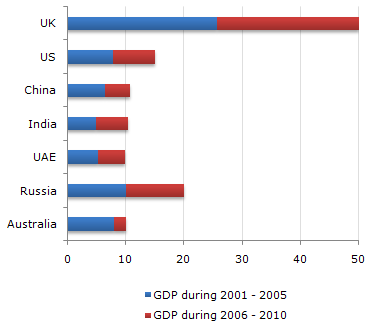# Data Interpretation - Bar Charts

The following bar chart represents the GDP of different countries during the half decades 2001 - 2005 and 2006 - 2010. All figures are in Rs. billion.

GDP of Various Countries1.

Which of the countries listed below accounts for the maximum GDP during the half decade 2006 to 2010 ?

 A. UAE B. US C. India D. China

Explanation:

It's clear that US is the highest amongst the given options.

2.

The GDP of UAE is what fraction of GDP of the UK for the decade (approximately) ?

 A. (1/4)th B. (1/5)th C. (1/6)th D. Data inadequate

Explanation:

(10/50) = 20% = (1/5)th

3.

Which of the countries listed below accounts for the highest GDP during the half decade 2001 to 2005 ?

 A. Russia B. China C. India D. UAE

Explanation:

It's clear that Russia is the answer.

4.

Out of every Rs. 10,000 spent during the decade 2001 - 2010 approximately how much was the GDP of Russia during the half decade 2001 - 2005 ?

 A. Rs. 700 B. Rs. 1,400 C. Rs. 2,800 D. None of these